# Classify Images Using Convolutional Neural Networks & Python

Build your own CNN using Keras

In this article I will show you how to create your very own Convolutional Neural Network (CNN) to classify images using the Python programming language and it’s library keras !

If you prefer not to read this article and would like a video representation of it, you can check out the video below. It goes through everything in this article with a little more detail and will help make it easy for you to start programming your own Convolutional Neural Network (CNN) model even if you don’t have the programming language Python installed on your computer. Or you can use both the video and this article as supplementary materials for learning about CNN’s!

# Start Programming:

`# Description: This programs classifies images`

Next, I need to install the dependencies / packages. If you don’t already have these packages installed, run the following command in your terminal, command prompt or, Google Colab website (depending on where you have your python programming language installed).

`pip install tensorflow keras numpy skimage matplotlib`

Import the libraries.

`import tensorflow as tffrom tensorflow import kerasfrom keras.models import Sequentialfrom keras.layers import Dense, Flatten, Conv2D, MaxPooling2D, Dropoutfrom tensorflow.keras import layersfrom keras.utils import to_categoricalimport numpy as npimport matplotlib.pyplot as pltplt.style.use('fivethirtyeight')`

Next, load the data set into the variables `x_train` (the variable that contains the images to train on) , `y_train` (the variable that contains the labels of the images in the training set), `x_test` (the variable that contains the images to test on), and the `y_test` (the variable that contains the labels of the images in the test set).

`#Load the datafrom keras.datasets import cifar10(x_train, y_train), (x_test, y_test) = cifar10.load_data()`

# Explore The Data

`#Print the data type of x_trainprint(type(x_train))#Print the data type of y_trainprint(type(y_train))#Print the data type of x_testprint(type(x_test))#Print the data type of y_testprint(type(y_test))`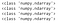The data types of the train & test data sets are numpy arrays

Get the shape of the `x_train` , `y_train` , `x_test` and `y_test` data. You will notice that the shape of the `x_train` data set is a 4-Dimensional array with 50,000 rows of 32 x 32 pixel image with depth = 3 (RGB) where R is Red, G is Green, and B is Blue. The `y_train` data shape is a 2-Dimensional array with 50,000 rows and 1 column. The shape of the `x_test` data set is a 4-Dimensional array with 10,000 rows of 32 x 32 pixel image with depth = 3 (RGB). The `y_test` data shape is a 2-Dimensional array with 10,000 rows and 1 column.

`#Get the shape of x_trainprint('x_train shape:', x_train.shape)#Get the shape of y_trainprint('y_train shape:', y_train.shape)#Get the shape of x_trainprint('x_test shape:', x_test.shape)#Get the shape of y_trainprint('y_test shape:', y_test.shape)`

Take a look at the first image (at index=0) in the training data set as a numpy array. This shows the image as a series of pixel values.

`index = 0x_train[index]`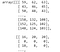Sample of the image as an array, these are the pixel (RGB) values

Show the image as an image instead of a series of pixel values using matplotlib.

`img = plt.imshow(x_train[index])`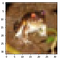The image as an image (this is an 32x32 image of a frog)

Print the label of the image. Notice the label printed was the number 6 this corresponds to the frog label.

`print('The image label is: ', y_train[index])`

Show the label classification in relation to the number.

`classification = ['airplane', 'automobile', 'bird', 'cat', 'deer', 'dog', 'frog', 'horse', 'ship', 'truck']#Print the image classprint('The image class is: ', classification[y_train[index]])`

Use One-Hot Encoding to convert the labels into a set of 10 numbers to input into the neural network. The numbers of course corresponds with the number of labels to classify the images.

`y_train_one_hot = to_categorical(y_train)y_test_one_hot = to_categorical(y_test)`

Print all of the new labels in the training data set.

`print(y_train_one_hot)`

Print an example of the new labels using the first image in the training data set.

NOTE: The label 6 = [0,0,0,0,0,0,1,0,0,0]

`print('The one hot label is:', y_train_one_hot)`

Normalize the pixels in the images to be a value between 0 and 1 , they are normally values between 0 and 255, doing this will help the neural network.

`x_train = x_train / 255x_test = x_test / 255`

# Build The Convolution Neural Network Model

`model = Sequential()`

Next we add the first layer, a convolution layer to extract features from the input image, and create 32 5 x 5 ReLu convoluted features also known as feature maps. Since this is the first layer we must input the dimension shape which is a 32 x 32 pixel image with depth = 3 (RGB).

`model.add(Conv2D(32, (5, 5), activation='relu', input_shape=(32,32,3)))`

The next layer will be a pooling layer with a 2 x 2 pixel filter to get the max element from the feature maps. This reduces the dimension of the feature maps by half and is also known as sub sampling.

`model.add(MaxPooling2D(pool_size=(2, 2)))`

Create one more convolution layer and pooling layer like before, but without the `input_shape`.

`model.add(Conv2D(64, (5, 5), activation='relu'))model.add(MaxPooling2D(pool_size=(2, 2)))`

Add a flattening layer, to reduce the image to a linear array also known as a one 1-Dimension vector to feed into and connect with the neural network.

`model.add(Flatten())`

Now create a neural network where the first layer has 1000 neurons and the activation function ReLu.

`model.add(Dense(1000, activation='relu'))`

Add a drop out layer with 50% drop out.

`model.add(Dropout(0.5))`

Now create a neural network where the first layer has 500 neurons and the activation function ReLu.

`model.add(Dense(500, activation='relu'))`

Add a drop out layer with 50% drop out.

`model.add(Dropout(0.5))`

Now create a neural network where the first layer has 250 neurons and the activation function ReLu.

`model.add(Dense(250, activation='relu'))`

Create the last layer of this neural network with 10 neurons (one for each label) using the softmax function.

`model.add(Dense(10, activation='softmax'))`

So the CNN should look like below when put together.

`model = Sequential()model.add(Conv2D(32, (5, 5), activation='relu', input_shape=(32,32,3)))model.add(MaxPooling2D(pool_size=(2, 2)))model.add(Conv2D(64, (5, 5), activation='relu'))model.add(MaxPooling2D(pool_size=(2, 2)))model.add(Flatten())model.add(Dense(1000, activation='relu'))model.add(Dropout(0.5))model.add(Dense(500, activation='relu'))model.add(Dropout(0.5))model.add(Dense(250, activation='relu'))model.add(Dense(10, activation='softmax'))`

Compile the model. Give it the `categorical_crossentropy` loss function which is used for classes greater than 2, the adam optimizer, and the accuracy of the model.

`model.compile(loss='categorical_crossentropy',               optimizer='adam',              metrics=['accuracy'])`

Train the model using the `fit()` method, which is another word for train. We will train the model on the training data with batch size =256, epochs =10, and split the data into training on 80% of the data and using the other 20% as validation. Training may take some time to finish.

Batch: Total number of training examples present in a single batch

Epoch:The number of iterations when an ENTIRE dataset is passed forward and backward through the neural network only ONCE.

Fit: Another word for train

`hist = model.fit(x_train, y_train_one_hot,            batch_size=256, epochs=10, validation_split=0.2 )`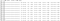Sample of training & model showing accuracy score of 71.22% on training data

# Get The Models Metrics

`model.evaluate(x_test, y_test_one_hot)`

Visualize the models accuracy for both the training and validation data.

`#Visualize the models accuracyplt.plot(hist.history['accuracy'])plt.plot(hist.history['val_accuracy'])plt.title('Model accuracy')plt.ylabel('Accuracy')plt.xlabel('Epoch')plt.legend(['Train', 'Val'], loc='upper left')plt.show()`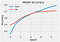A visualization of the models accuracy for training and validation set

Visualize the models loss for both the training and validation data.

`#Visualize the models lossplt.plot(hist.history['loss'])plt.plot(hist.history['val_loss'])plt.title('Model loss')plt.ylabel('Loss')plt.xlabel('Epoch')plt.legend(['Train', 'Val'], loc='upper right')plt.show()`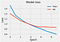A visualization of the models loss for training and validation set

# Test The Model

`#Load the datafrom google.colab import files # Use to load data on Google Colabuploaded = files.upload() # Use to load data on Google Colabnew_image = plt.imread("cat.4015.jpg") #Read in the image (3, 14, 20)`

`img = plt.imshow(new_image)`

Resize the image to a 32 x 32 pixel image with depth = 3, and show the image.

`from skimage.transform import resizeresized_image = resize(new_image, (32,32,3))img = plt.imshow(resized_image)`

Get the predictions for each class and store it into a variable.

`predictions = model.predict(np.array( [resized_image] ))`

Show the predictions

`predictions`

Sort the predictions from least to greatest such that the highest probability is at index=9 and the lowest probability is at index = 0.

`list_index = [0,1,2,3,4,5,6,7,8,9]x = predictionsfor i in range(10):  for j in range(10):    if x[list_index[i]] > x[list_index[j]]:      temp = list_index[i]      list_index[i] = list_index[j]      list_index[j] = temp#Show the sorted labels in order from highest probability to lowestprint(list_index)`

Print the first 5 most likely classes and the corresponding probability.

`i=0for i in range(5):  print(classification[list_index[i]], ':', round(predictions[list_index[i]] * 100, 2), '%')`

Looks like this model was able to accurately classify the given image as a cat with 50.65 % likelihood. This is good, but from the metrics that were gathered earlier, this model isn’t very accurate, it has an accuracy of only 70.43%. So although the accuracy is better than guessing it could still benefit greatly with possibly more training data, and some fine tuning of the model.

Let’s save this model for later use.

`#To save this model model.save('my_model.h5')`

To load this model later, without having to train a brand new one, you can do the following.

`#To load this modelfrom keras.models import load_modelmodel = load_model('my_model.h5')`

You can see the video above for how I coded this program and code along with me with a few more detailed explanations, or you can just click the YouTube link here.

If you are also interested in reading more on machine learning to immediately get started with problems and examples then I strongly recommend you check out Hands-On Machine Learning with Scikit-Learn and TensorFlow: Concepts, Tools, and Techniques to Build Intelligent Systems. It is a great book for helping beginners learn how to write machine learning programs, and understanding machine learning concepts.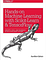Hands-On Machine Learning with Scikit-Learn and TensorFlow: Concepts, Tools, and Techniques to Build Intelligent Systems

# Resources:

 Keras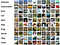Images and their labels from the CIFAR-10 dataset. Image taken from https://www.cs.toronto.edu/~kriz/cifar.html

Written by

Written by